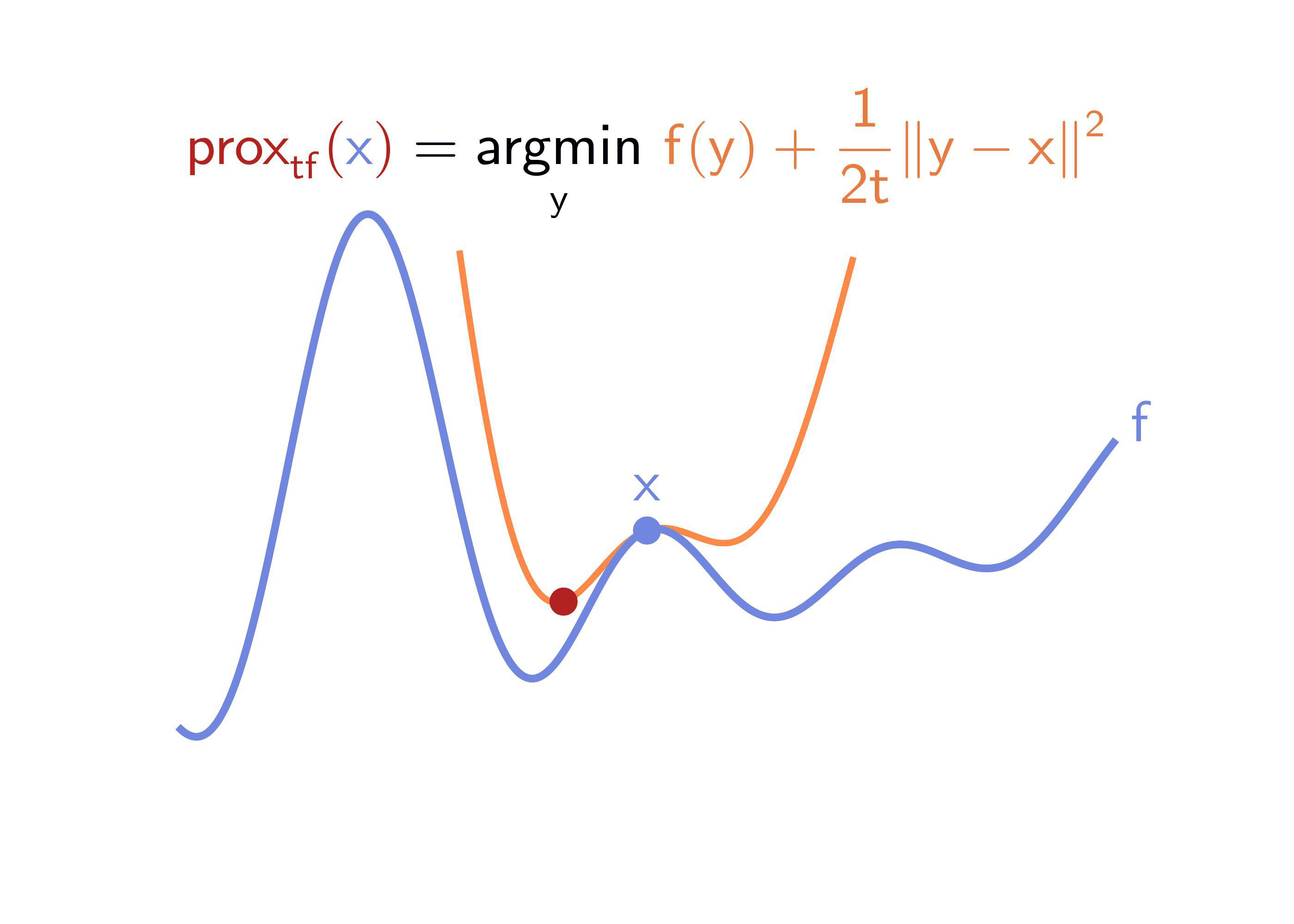# A Hamilton-Jacobi-based Proximal OperatorStanley Osher, Howard Heaton, and Samy Wu Fung

Summary

We give a formula for estimating proximal operators from (possibly noisy) observations of objective function values.

Key Steps

• Sample points $$\mathsf{y^i}$$ (via a Gaussian) about the input $$\mathsf{x}$$
• Evaluate function $$\mathsf{f}$$ at each point $$\mathsf{y^i}$$
• Estimate proximal by using softmax to combine the values for $$\mathsf{f(y^i)}$$ and $$\mathsf{y^i}$$Abstract

First-order optimization algorithms are widely used today. Two standard building blocks in these algorithms are proximal operators (proximals) and gradients. Although gradients can be computed for a wide array of functions, explicit proximal formulas are known for only limited classes of functions. We provide an algorithm, HJ-Prox, for accurately approximating such proximals. This is derived from a collection of relations between proximals, Moreau envelopes, Hamilton–Jacobi (HJ) equations, heat equations, and Monte Carlo sampling. In particular, HJ-Prox smoothly approximates the Moreau envelope and its gradient. The smoothness can be adjusted to act as a denoiser. Our approach applies even when functions are accessible only by (possibly noisy) black box samples. We show that HJ-Prox is effective numerically via several examples.

Citation

@article{osher2023hamilton,
title={{A Hamilton-Jacobi-based proximal operator}},
author={Osher, Stanley and Heaton, Howard and Fung, Samy Wu},
journal={{Proceedings of the National Academy of Sciences}},
year={2023},
volume={120},
number={14}
}